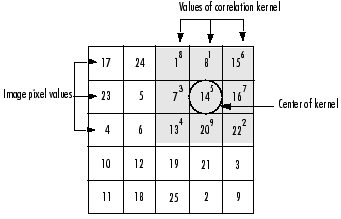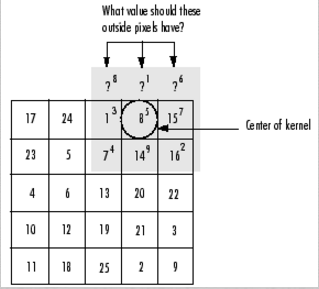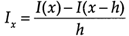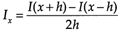# 图像处理卷积

## 【图像处理】卷积算法

2016-06-06 17:34:31 xueyedie1234 阅读数 24422
• ###### MATLAB图像处理

介绍MATLAB提供的图像处理功能，包括图像基础知识、图像合成、空间变换、邻域和块处理、局部滤波、正交变换、数学形态学、图像分析、图像增强、图像恢复、图像分割、图像配准和图像三维重建等内容。

47课时 342分钟 212人学习 苏金明
免费试看

### 文章目录

# 一、 什么是卷积？       在图像处理中，卷积操作指的是使用一个卷积核对图像中的每个像素进行一系列操作。       卷积核（算子）是用来做图像处理时的矩阵,图像处理时也称为掩膜，是与原图像做运算的参数。卷积核通常是一个四方形的网格结构（例如3*3的矩阵或像素区域），该区域上每个方格都有一个权重值。       使用卷积进行计算时，需要将卷积核的中心放置在要计算的像素上，一次计算核中每个元素和其覆盖的图像像素值的乘积并求和，得到的结构就是该位置的新像素值。       以下两个算子中演示了具体的卷积计算过程。
# 二、 相关算子 定义：![这里写图片描述](http://img.blog.csdn.net/20160603125126517) 即![这里写图片描述](http://img.blog.csdn.net/20160603125155767)，其中ｈ称为相关核(Kernel). 　　　　　　　　 步骤：
• 1）滑动核，使其中心位于输入图像g的（i，j）像素上
• 2）利用上式求和，得到输出图像的（i，j）像素值
• 3）充分上面操纵，直到求出输出图像的所有像素值

【例】
原始像素矩阵为：
\begin{bmatrix}
17 & 24 & 1 & 8 & 15 \
23 & 5 & 7 & 14 & 16 \
4 & 6 & 13 & 20 & 22 \
10 & 12 & 19 & 21 & 3\
11 & 18 & 25 & 2 & 9
\end{bmatrix}

\begin{bmatrix}
8 & 1 & 6 \
3 & 5 & 7 \
4 & 9 & 2
\end{bmatrix}# 三、 卷积算子• 1）将核围绕中心旋转180度
• 2）滑动核，使其中心位于输入图像g的（i，j）像素上
• 3）利用上式求和，得到输出图像的（i，j）像素值
• 4）充分上面操纵，直到求出输出图像的所有像素值
例：计算输出图像的（2，4）元素=12+89+154+77+145+163+136+201+22*8=575
如图所示：# 四、 边缘效应       当对图像边缘的进行滤波时，核的一部分会位于图像边缘外面。 ![这里写图片描述](http://img.blog.csdn.net/20160603143253118) 常用的策略包括： - 1）使用常数填充：imfilter默认用0填充，这会造成处理后的图像边缘是黑色的。 - 2）复制边缘像素：I3 = imfilter(I,h,'replicate'); ![这里写图片描述](http://img.blog.csdn.net/20160603143353525)
# 五、 常用的卷积核及其用途
• 1）低通滤波器（常用于计算模糊后的效果）\begin{bmatrix}
1/9 & 1/9 & 1/9 \
1/9 & 1/9 & 1/9 \
1/9 & 1/9 & 1/9
\end{bmatrix}
\begin{bmatrix}
1/10 & 1/10 & 1/10 \
1/10 & 2/10 & 1/10 \
1/10 & 1/10 & 1/10
\end{bmatrix}
\begin{bmatrix}
1/16 & 2/16 & 1/16 \
2/16 & 4/16 & 2/16 \
1/16 & 2/16 & 1/16
\end{bmatrix}

• 2）高斯滤波器（常用于计算高斯模糊后的效果）
高斯模糊的卷积核也是一个正方形的滤波核，其中每个元素通过以下公式计算得出：
$G(x,y)=\frac{1}{2πσ^{2}}·e^{\frac{x^{2}+y^{2}}{2σ^{2}}}$
该公式中σ是标准方差（一般取值为1），x和y分别对应了当前位置到卷积核中心的整数距离。通过这个公式，就可以计算出高斯核中每个位置对应的值。为了保证滤波后的图像不会变暗，需要对高斯核中的权重进行归一化。

• 3）边缘检测（常用于计算图像边缘或者说梯度值）
\begin{bmatrix}
-1 & 0 & -1 \
0 & 4 & 0 \
-1 & 0 & -1
\end{bmatrix}

# 六、 一个例子——使用卷积实现模糊效果       我们将对下面这张图进行模糊处理： ![这里写图片描述](http://img.blog.csdn.net/20160606172529227)       以下为compute shader中关于卷积处理的代码：
[numthreads(32,32,1)]
void Dim_Main (uint3 id : SV_DispatchThreadID)
{

float sumR = 0;
float sumG = 0;
float sumB = 0;
float sumA = 0;
for (int i = -1; i <= 1; i++)
{
for (int j = -1; j <= 1; j++)
{
sumR += texBuffer[(id.x+i)*texWidth+(id.y+j)].r * convolutionTempBuffer[(i+1)*3+(j+1)];
sumG += texBuffer[(id.x+i)*texWidth+(id.y+j)].g * convolutionTempBuffer[(i+1)*3+(j+1)];
sumB += texBuffer[(id.x+i)*texWidth+(id.y+j)].b * convolutionTempBuffer[(i+1)*3+(j+1)];
sumA += texBuffer[(id.x+i)*texWidth+(id.y+j)].a * convolutionTempBuffer[(i+1)*3+(j+1)];
}
}

texBuffer[id.x*texWidth+id.y].r = sumR;
texBuffer[id.x*texWidth+id.y].g = sumG;
texBuffer[id.x*texWidth+id.y].b = sumB;
texBuffer[id.x*texWidth+id.y].a = sumA;

Result[id.xy] = float4(sumR, sumG, sumB, sumA);
}图中可以明显的看到左右两边有明显的黑色线条，原图中是没有这样的黑色的，产生这种效果的原因是本文中之前提到过的边缘效应。下面我将修改一部分代码去除边缘效应带来的影响，这里使用的是相邻像素的值方法。

[numthreads(32,32,1)]
void Dim_Main (uint3 id : SV_DispatchThreadID)
{

float sumR = 0;
float sumG = 0;
float sumB = 0;
float sumA = 0;
for (int i = -1; i <= 1; i++)
{
for (int j = -1; j <= 1; j++)
{
if((id.x+i)*texWidth+(id.y+j)>texWidth*texWidth-1)
{
sumR += texBuffer[(id.x+i)*texWidth+(id.y+j)-texWidth].r * convolutionTempBuffer[(i+1)*3+(j+1)];
sumG += texBuffer[(id.x+i)*texWidth+(id.y+j)-texWidth].g * convolutionTempBuffer[(i+1)*3+(j+1)];
sumB += texBuffer[(id.x+i)*texWidth+(id.y+j)-texWidth].b * convolutionTempBuffer[(i+1)*3+(j+1)];
sumA += texBuffer[(id.x+i)*texWidth+(id.y+j)-texWidth].a * convolutionTempBuffer[(i+1)*3+(j+1)];
}

sumR += texBuffer[(id.x+i)*texWidth+(id.y+j)].r * convolutionTempBuffer[(i+1)*3+(j+1)];
sumG += texBuffer[(id.x+i)*texWidth+(id.y+j)].g * convolutionTempBuffer[(i+1)*3+(j+1)];
sumB += texBuffer[(id.x+i)*texWidth+(id.y+j)].b * convolutionTempBuffer[(i+1)*3+(j+1)];
sumA += texBuffer[(id.x+i)*texWidth+(id.y+j)].a * convolutionTempBuffer[(i+1)*3+(j+1)];
}
}

texBuffer[id.x*texWidth+id.y].r = sumR;
texBuffer[id.x*texWidth+id.y].g = sumG;
texBuffer[id.x*texWidth+id.y].b = sumB;
texBuffer[id.x*texWidth+id.y].a = sumA;

Result[id.xy] = float4(sumR, sumG, sumB, sumA);
}可以看到，图中左边的黑色线条已经被滤除，右边也可以采用类似的方法来剔除。实际使用中，也可以根据情况使用纯色来做剔除，这样可以节省部分效率，如下图中我使用的是纯白色来剔除边缘效应。## 图像处理算法——卷积

2018-06-10 21:49:47 xiansong1005 阅读数 2762
• ###### MATLAB图像处理

介绍MATLAB提供的图像处理功能，包括图像基础知识、图像合成、空间变换、邻域和块处理、局部滤波、正交变换、数学形态学、图像分析、图像增强、图像恢复、图像分割、图像配准和图像三维重建等内容。

47课时 342分钟 212人学习 苏金明
免费试看

# 一、 什么是卷积？

在图像处理中，卷积操作指的是使用一个卷积核对图像中的每个像素进行一系列操作。
卷积核（算子）是用来做图像处理时的矩阵,图像处理时也称为掩膜，是与原图像做运算的参数。卷积核通常是一个四方形的网格结构（例如3*3的矩阵或像素区域），该区域上每个方格都有一个权重值。
使用卷积进行计算时，需要将卷积核的中心放置在要计算的像素上，一次计算核中每个元素和其覆盖的图像像素值的乘积并求和，得到的结构就是该位置的新像素值。
以下两个算子中演示了具体的卷积计算过程。

# 二、 相关算子，其中ｈ称为相关核(Kernel).

• 1）滑动核，使其中心位于输入图像g的（i，j）像素上
• 2）利用上式求和，得到输出图像的（i，j）像素值
• 3）充分上面操纵，直到求出输出图像的所有像素值

【例】
原始像素矩阵为：

17234101124561218171319258142021215162239

卷积模板h为：

834159672# 三、 卷积算子- 1）将核围绕中心旋转180度
- 2）滑动核，使其中心位于输入图像g的（i，j）像素上
- 3）利用上式求和，得到输出图像的（i，j）像素值
- 4）充分上面操纵，直到求出输出图像的所有像素值# 四、 边缘效应

当对图像边缘的进行滤波时，核的一部分会位于图像边缘外面。- 1）使用常数填充：imfilter默认用0填充，这会造成处理后的图像边缘是黑色的。
- 2）复制边缘像素：I3 = imfilter(I,h,’replicate’);# 五、 常用的卷积核及其用途

• 1）低通滤波器（常用于计算模糊后的效果）
1/91/91/91/91/91/91/91/91/9[1/91/91/91/91/91/91/91/91/9]

1/101/101/101/102/101/101/101/101/10[1/101/101/101/102/101/101/101/101/10]

1/162/161/162/164/162/161/162/161/16[1/162/161/162/164/162/161/162/161/16]
• 2）高斯滤波器（常用于计算高斯模糊后的效果）
高斯模糊的卷积核也是一个正方形的滤波核，其中每个元素通过以下公式计算得出：
G(x,y)=12πσ2ex2+y22σ2G(x,y)=12πσ2·ex2+y22σ2
该公式中σ是标准方差（一般取值为1），x和y分别对应了当前位置到卷积核中心的整数距离。通过这个公式，就可以计算出高斯核中每个位置对应的值。为了保证滤波后的图像不会变暗，需要对高斯核中的权重进行归一化。

• 3）边缘检测（常用于计算图像边缘或者说梯度值）

101040101[−10−1040−10−1]

## 图像处理中卷积的作用

2019-05-30 23:43:32 Europe233 阅读数 911
• ###### MATLAB图像处理

介绍MATLAB提供的图像处理功能，包括图像基础知识、图像合成、空间变换、邻域和块处理、局部滤波、正交变换、数学形态学、图像分析、图像增强、图像恢复、图像分割、图像配准和图像三维重建等内容。

47课时 342分钟 212人学习 苏金明
免费试看

$\quad$图像处理之中，有很多算法是基于卷积的：图像滤波、边缘提取都是对整幅图像做了一个卷积。由于图像是离散的，所以实际上是图像矩阵和一个卷积模板做了卷积操作。通常滤波的卷积模板权值和为1（因为滤波其实是在取均值），边缘提取的模板权值和为0（因为是在做差分）。

$\quad$为什么会用到卷积呢？因为在这里用卷积，会具有空不变性，或者说，具有一种空间上的平移不变性。假设原图像为$f(i,j)$,卷积模板是$S(i,j)$,以$O(i,j)$表示$(i,j)$的领域,卷积后的图像是$g(i,j)$:
$g(i,j)={\sum\sum}_{(t,k)\in O(i,j)}f(t,k)S(i-t,j-k)$$\quad$上面提到的空不变性是指：对于原图像中的每个像素点，都进行了同样的操作，都以同一个卷积模板套在这个像素点上，对其领域内所有像素点的灰度值进行加权求和。当中心像素点滑动，卷积模板也会跟着滑动，始终保证中心像素点在卷积模板的中心。

$\quad$再说一些关于卷积的东西，上面的卷积具有空不变性，如果卷积是这个形式：
$\int_{t_0}^{t_e} f(s)g(t-s)ds$其中的t代表时间，就表示这个形式的卷积具有时不变性，或者有一种时间上的平移不变性。对于函数f的所有点，它们在时间维度上受到了同样的操作。这一点很难表达，可以看这个链接中的例子：

【如何通俗易懂地解释卷积？ - palet的回答 - 知乎
$\quad$这个回答中对于“卷积”一词的理解也非常有趣：“先卷再积”，实在生动形象。

## 图像处理之理解卷积

2011-12-04 10:42:40 jia20003 阅读数 103987
• ###### MATLAB图像处理

介绍MATLAB提供的图像处理功能，包括图像基础知识、图像合成、空间变换、邻域和块处理、局部滤波、正交变换、数学形态学、图像分析、图像增强、图像恢复、图像分割、图像配准和图像三维重建等内容。

47课时 342分钟 212人学习 苏金明
免费试看

f(x) =  - 其中C(k)代表卷积操作数，g(i)代表样本数据, f(x)代表输出结果。1.      读取源图像像素

2.      应用卷积操作数矩阵产生目标图像

3.      对目标图像进行归一化处理

4.      处理边界像素// red color

out3DData[row][col] =in3DData[row][col] +

in3DData[row-1][col] +

in3DData[row+1][col] +

in3DData[row][col-1] +

in3DData[row-1][col-1] +

in3DData[row+1][col-1] +

in3DData[row][col+1] +

in3DData[row-1][col+1] +

in3DData[row+1][col+1];

// green color

out3DData[row][col] =in3DData[row][col] +

in3DData[row-1][col] +

in3DData[row+1][col] +

in3DData[row][col-1] +

in3DData[row-1][col-1] +

in3DData[row+1][col-1] +

in3DData[row][col+1] +

in3DData[row-1][col+1] +

in3DData[row+1][col+1];

// blue color

out3DData[row][col] =in3DData[row][col] +

in3DData[row-1][col] +

in3DData[row+1][col] +

in3DData[row][col-1] +

in3DData[row-1][col-1] +

in3DData[row+1][col-1] +

in3DData[row][col+1] +

in3DData[row-1][col+1] +

in3DData[row+1][col+1];

// find the peak data frominput and output pixel data.

int inpeak = 0;

int outPeak = 0;

for(int row=0; row<srcH; row++) {

for(int col=0; col<srcW; col++) {

if(inpeak < in3DData[row][col]) {

inpeak = in3DData[row][col];

}

if(inpeak < in3DData[row][col]) {

inpeak = in3DData[row][col];

}

if(inpeak < in3DData[row][col]) {

inpeak = in3DData[row][col];

}

if(outPeak < out3DData[row][col]) {

outPeak = out3DData[row][col];

}

if(outPeak < out3DData[row][col]) {

outPeak = out3DData[row][col];

}

if(outPeak < out3DData[row][col]) {

outPeak = out3DData[row][col];

}

}

}

// normalization

double outputScale = ((double) inpeak) / ((double)outPeak);

for(int row=0; row<srcH; row++) {

for(int col=0; col<srcW; col++) {

out3DData[row][col] = (int)(outputScale * out3DData[row][col]);

out3DData[row][col] = (int)(outputScale * out3DData[row][col]);

out3DData[row][col] = (int)(outputScale * out3DData[row][col]);

}

}

视频教程 - 《数字图像处理-空间域卷积》

## 图像处理（卷积）

2018-08-24 22:41:48 weixin_42797060 阅读数 2422
• ###### MATLAB图像处理

介绍MATLAB提供的图像处理功能，包括图像基础知识、图像合成、空间变换、邻域和块处理、局部滤波、正交变换、数学形态学、图像分析、图像增强、图像恢复、图像分割、图像配准和图像三维重建等内容。

47课时 342分钟 212人学习 苏金明
免费试看

# 图像处理－线性滤波－1 基础（相关算子、卷积算子、边缘效应）

## 1.相关算子（Correlation Operator)

步骤：

1）滑动核，使其中心位于输入图像g的（i，j）像素上

2）利用上式求和，得到输出图像的（i，j）像素值

3）充分上面操纵，直到求出输出图像的所有像素值

例：

A = [17  24      15            h = [8     6
23      14  16                         7
13  20  22                         2]
10  12  19  21
11  18  25     9]

Matlab 函数：imfilter(A,h)

## 2.卷积算子（Convolution)

步骤：

1）将核围绕中心旋转180度

2）滑动核，使其中心位于输入图像g的（i，j）像素上

3）利用上式求和，得到输出图像的（i，j）像素值

4）充分上面操纵，直到求出输出图像的所有像素值

Matlab 函数：Matlab 函数：imfilter(A,h,’conv’)% imfilter默认是相关算子，因此当进行卷积计算时需要传入参数’conv’

## 3.边缘效应

1）使用常数填充：imfilter默认用0填充，这会造成处理后的图像边缘是黑色的。

2）复制边缘像素：I3 = imfilter(I,h,’replicate’);

## 4.常用滤波

fspecial函数可以生成几种定义好的滤波器的相关算子的核。

 1 2 3 4 5 I = imread(’moon.tif’); h = fspecial(’unsharp’); I2 = imfilter(I,h); imshow(I), title(’Original Image’) figure, imshow(I2), title(’Filtered Image’)

# 图像处理－线性滤波－2 图像微分（1、2阶导数和拉普拉斯算子）

## 1 一阶导数，前向差分 forward differencing                  （1.2），中心差分 central differencing                     （1.3）

1）前向差分的Matlab实现

 1 2 3 4 5 6 7 8 9 10 11 12 13 14 15 16 17 18 19 20 21 22 23 24 25 26 27 function dimg = mipforwarddiff(img,direction) % MIPFORWARDDIFF     Finite difference calculations  % %   DIMG = MIPFORWARDDIFF(IMG,DIRECTION) % %  Calculates the forward-difference for a given direction %  IMG       : input image %  DIRECTION : ’dx’ or 'dy' %  DIMG      : resultant image % %   See also MIPCENTRALDIFF MIPBACKWARDDIFF MIPSECONDDERIV %   MIPSECONDPARTIALDERIV    %   Omer Demirkaya, Musa Asyali, Prasana Shaoo, ... 9/1/06 %   Medical Image Processing Toolbox    imgPad = padarray(img,[1 1],’symmetric’,'both');%将原图像的边界扩展 [row,col] = size(imgPad); dimg = zeros(row,col); switch (direction)    case 'dx',    dimg(:,1:col-1) = imgPad(:,2:col)-imgPad(:,1:col-1);%x方向差分计算， case 'dy',    dimg(1:row-1,:) = imgPad(2:row,:)-imgPad(1:row-1,:);  otherwise, disp(’Direction is unknown’); end; dimg = dimg(2:end-1,2:end-1);

2）中心差分的Matlab实现

 1 2 3 4 5 6 7 8 9 10 11 12 13 14 15 16 17 18 19 20 21 22 23 24 25 26 27 28 function dimg = mipcentraldiff(img,direction) % MIPCENTRALDIFF     Finite difference calculations  % %   DIMG = MIPCENTRALDIFF(IMG,DIRECTION) % %  Calculates the central-difference for a given direction %  IMG       : input image %  DIRECTION : ’dx’ or 'dy' %  DIMG      : resultant image % %   See also MIPFORWARDDIFF MIPBACKWARDDIFF MIPSECONDDERIV %   MIPSECONDPARTIALDERIV    %   Omer Demirkaya, Musa Asyali, Prasana Shaoo, ... 9/1/06 %   Medical Image Processing Toolbox    img = padarray(img,[1 1],’symmetric’,'both'); [row,col] = size(img); dimg = zeros(row,col); switch (direction)     case 'dx',         dimg(:,2:col-1) = (img(:,3:col)-img(:,1:col-2))/2;     case 'dy',         dimg(2:row-1,:) = (img(3:row,:)-img(1:row-2,:))/2;     otherwise,         disp(’Direction is unknown’); end dimg = dimg(2:end-1,2:end-1);
 1 

 1 2 I = imread(’coins.png’); figure; imshow(I); Id = mipforwarddiff(I,’dx’); figure, imshow(Id);

原图像                                                   x方向1阶导数

Matlab函数

2）quiver：以箭头形状绘制梯度。注意放大下面最右侧图可看到箭头，由于这里计算横竖两个方向的梯度，因此箭头方向都是水平或垂直的。

 1 2 3 4 5 I = double(imread('coins.png')); [dx,dy]=gradient(I); magnitudeI=sqrt(dx.^2+dy.^2); figure;imagesc(magnitudeI);colormap(gray);%梯度幅值 hold on;quiver(dx,dy);%叠加梯度方向

梯度幅值                                   梯度幅值+梯度方向

## 3.1 普拉斯算子（laplacian operator）

2）因此，二阶差分为

### 3.1.2 应用

Matlab里有两个函数

1）del2

2）fspecial：图像处理中一般利用Matlab函数fspecial

h = fspecial(‘laplacian’, alpha) returns a 3-by-3 filter approximating the shape of the two-dimensional Laplacian operator.
The parameter alpha controls the shape of the Laplacian and must be in the range 0.0 to 1.0. The default value for alpha is 0.2.

### 3.1.3 资源

sift算法

Sift算法就是用不同尺度（标准差）的高斯函数对图像进行平滑，然后比较平滑后图像的差别，

sift可以同时处理亮度，平移，旋转，尺度的变化，利用特征点来提取特征描述符，最后在特征描述符之间寻找匹配

1构建尺度空间，检测极值点，获得尺度不变性

2特征点过滤并进行经确定位，剔除不稳定的特征点

3 在特征点处提取特征描述符，为特征点分配方向直

4声称特征描述子，利用特征描述符寻找匹配点

5计算变换参数

L(x,y,delta)=G(x,y,e)*i(x,y)

G(x,y,e) = [1/2*pi*e^2] * exp[ -(x^2 + y^2)/2e^2]

D(x,y,e)=(G(x,y,ke)-G(x,y,e))*i(x,y)

=L(x,y,ke)-L(x,y,e)

(为避免遍历每个像素点)

L(x,y,e) = G(x,y,e)*I(x,y)

图像金字塔的构建：图像金字塔共O组，每组有S层，下一组的图像由上一组图像降采样得到、

在尺度空间建立完毕后，为了能够找到稳定的关键点，采用高斯差分的方法来检测那些在局部位置的极值点，即采用俩个相邻的尺度中的图像相减，即公式定义为：
D(x,y,e) = ((G(x,y,ke) - G(x,y,e)) * I(x,y)
= L(x,y,ke) - L(x,y,e)
咱们再来具体阐述下构造D(x,y,e)的详细步骤：
1、首先采用不同尺度因子的高斯核对图像进行卷积以得到图像的不同尺度空间，将这一组图像作为金子塔图像的第一层。
2、接着对第一层图像中的2倍尺度图像（相对于该层第一幅图像的2倍尺度）以2倍像素距离进行下采样来得到金子塔图像的第二层中的第一幅图像，对该图像采用不同尺度因子的高斯核进行卷积，以获得金字塔图像中第二层的一组图像。
3、再以金字塔图像中第二层中的2倍尺度图像（相对于该层第一幅图像的2倍尺度）以2倍像素距离进行下采样来得到金字塔图像的第三层中的第一幅图像，对该图像采用不同尺度因子的高斯核进行卷积，以获得金字塔图像中第三层的一组图像。这样依次类推，从而获得了金字塔图像的每一层中的一组图像，
4、对上图得到的每一层相邻的高斯图像相减，就得到了高斯差分图像，如下述第一幅图所示。下述第二幅图中的右列显示了将每组中相邻图像相减所生成的高斯差分图像的结果，限于篇幅，图中只给出了第一层和第二层高斯差分图像的计算

——人（系统！）挨板子（脉冲！）以后，会有什么表现（输出！）？
——费话，疼呗！
——我问的是：会有什么表现？
——看疼到啥程度。像这无赖的体格，每天挨一个板子啥事都不会有，连哼一下都不可能，你也看到他那得意洋洋的嘴脸了（输出0）；如果一次连揍他十个板子，他可能会皱皱眉头，咬咬牙，硬挺着不哼
（输出1）；揍到二十个板子，他会疼得脸部扭曲，象猪似地哼哼（输出3）；揍到三十个板子，他可能会象驴似地嚎叫，一把鼻涕一把泪地求你饶他一命（输出5）；揍到四十个板子，他会大小便失禁，勉

——呜呼呀！这曲线象一座高山，弄不懂弄不懂。为啥那个无赖连挨了三十天大板却不喊绕命呀？
—— 呵呵，你打一次的时间间隔（Δτ=24小时）太长了，所以那个无赖承受的痛苦程度一天一利索，没有叠加，始终是一个常数；如果缩短打板子的时间间隔（建议 Δτ=0.5秒），那他的痛苦程度可就迅速叠加了；等到这无赖挨三十个大板（t=30）时，痛苦程度达到了他能喊叫的极限，会收到最好的惩戒效果，再多打就显示不出您的仁慈了。
——还是不太明白，时间间隔小，为什么痛苦程度会叠加呢？
——这与人（线性时不变系统）对板子（脉冲、输入、激励）的响应有关。什么是响应？人挨一个板子后，疼痛的感觉会在一天（假设的，因人而异）内慢慢消失（衰减），而不可能突然消失。这样一来，只要打板子的时间间隔很小，每一个板子引起的疼痛都来不及完全衰减，都会对最终的痛苦程度有不同的贡献：
t个大板子造成的痛苦程度=Σ(第τ个大板子引起的痛苦*衰减系数)
[衰减系数是（t-τ）的函数，仔细品味]

——拿人的痛苦来说卷积的事，太残忍了。除了人以外，其他事物也符合这条规律吗？
——呵呵，县令大人毕竟仁慈。其实除人之外，很多事情也遵循此道。好好想一想，铁丝为什么弯曲一次不折，快速弯曲多次却会轻易折掉呢？
——恩，一时还弄不清，容本官慢慢想来——但有一点是明确地——来人啊，将撒尿的那个无赖抓来，狠打40大板！

1。你信号来的时候正赶上人家”系统”的响应时间段吗？
2。就算赶上系统响应时间段，响应有多少？

*********拉普拉斯*********

“拉 普拉斯变换” 就相当于上述例子中把数转换成”指数” 的过程；进行了拉普拉斯变换之后，复杂的微分方程(对应于上例中”复杂”的乘法) 就变成了简单的代数方程，就象上例中”复杂”的乘法变成了简单的加减法。再把简单的代数方程的解反变换回去(就象把指数重新转换会一般的数一样)，就解决了原来那个复杂的微分方程。

1 。拉普拉斯变换之所以现在在电路中广泛应有，根本原因是电路中也广泛涉及了微分方程。
2。拉普拉斯变换与Z变换当然有紧密联系；其本质区别在于拉氏变换处理的是时间上连续的问题，Z变换处理的是时间上分立的问题。
Signals, Linear Systems, and Convolution

比如说你的老板命令你干活，你却到楼下打台球去了，后来被老板发现，他非常气愤，扇了你一巴掌（注意，这就是输入信号，脉冲），于是你的脸上会渐渐地（贱贱地）鼓起来一个包，你的脸就是一个系统，而鼓起来的包就是你的脸对巴掌的响应。
好，这样就和信号系统建立起来意义对应的联系。下面还需要一些假设来保证论证的严谨：假定你的脸是线性时不变系统，也就是说，无论什么时候老板打你一巴掌，打在你脸的同一位置（这似乎要求你的脸足够光滑，如果你说你长了很多青春痘，甚至整个脸皮处处连续处处不可导，那难度太大了，我就无话可说了），你的脸上总是会在相同的时间间隔内鼓起来一个相同高度的包来，并且假定以鼓起来的包的大小作为系统输出。好了，那么，下面可以进入核心内容——卷积了！
如果你每天都到楼下去打台球，那么老板每天都要扇你一巴掌，不过当老板打你一巴掌后，你5分钟就消肿了，所以时间长了，你甚至就适应这种生活了……如果有一天，老板忍无可忍，以0.5秒的间隔开始不间断的扇你的过程，这样问题就来了：第一次扇你鼓起来的包还没消肿，第二个巴掌就来了，你脸上的包就可能鼓起来两倍高，老板不断扇你，脉冲不断作用在你脸上，效果不断叠加了，这样这些效果就可以求和了，结果就是你脸上的包的高度岁时间变化的一个函数了（注意理解）！
如果老板再狠一点，频率越来越高，以至于你都辨别不清时间间隔了，那么，求和就变成积分了。可以这样理解，在这个过程中的某一固定的时刻，你的脸上的包的鼓起程度和什么有关呢？和之前每次打你都有关！但是各次的贡献是不一样的，越早打的巴掌，贡献越小，这就是说，某一时刻的输出是之前很多次输入乘以各自的衰减系数之后的叠加而形成某一点的输出，然后再把不同时刻的输出点放在一起，形成一个函数，这就是卷积。卷积之后的函数就是你脸上的包的大小随时间变化的函数。本来你的包几分钟就可以消肿，可是如果连续打，几个小时也消不了肿了，这难道不是一种平滑过程么？反映到公式上，f(a)就是第a个巴掌，g(x-a)就是第a个巴掌在x时刻的作用程度，乘起来再叠加就ok了，这就是卷积！
最后提醒各位，请勿亲身尝试……

3.1 一维卷积的定义

定义。这里参量x和积分变量α皆为实数；函数f和h可实可复。

1.1 一维傅立叶变换的定义与傅立叶积分定理

卷积公式是用来求随机变量和的密度函数(pdf)的计算公式。
定义式：
z(t)=x(t)*y(t)= ∫x(m)y(t-m)dm.
已知x,y的pdf,x(t),y(t).现在要求z=x+y的pdf. 我们作变量替显，令
z=x+y,m=x. 雅可比行列式=1.那么,t，m联合密度就是f(z,m)=x(m)y(z-m)*1. 这样，就可以很容易求Z的在（z,m)中边缘分布
即fZ(z)=∫x(m)y(z-m)dm….. 由于这个公式和x(t),y(t)存在一一对应的关系。为了方便，所以记 ∫x(m)y(z-m)dm=x(t)*y(t)

for(i=0; i<N; i++)
{
for(j=0; j<N; j++)
{
g[i*N+j]=exp(-((i-(N-1)/2)^2+(j-(N-1)/2)^2))/(2*delta^2));
sum += g[i*N+j];
}
}

N是滤波器的大小，delta自选